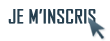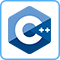IdentifiantMot de passe
Mot de passe oublié ?

Inscrivez-vous gratuitement
pour pouvoir participer, suivre les réponses en temps réel, voter pour les messages, poser vos propres questions et recevoir la newsletterDiscussion :

# Je ne comprend pas très bien les templates...

Sujet :

## C++

1.Je ne comprend pas très bien les templates...
Bonjour!

J'ai du resoudre l'exercice suivant:

" Créer une class Pile (template) [e.g. Pilha<Pair,10>p;]. La Pile doit être polymorfique, avoir une subclass Point de la class Pair. Les éléments de la class et de la subclass peuvent être insérés dans la Pile pp.

La class doit contenir seulement les suivants méthodes publiques:

- void push(C i)
- C pop()
- void printpile().

Je dois faire un programme pour insérer et rétirer d'une manière interactive des objects de la class Pair e de sa subclasse Point d'une pile de taille 10. Pair et Point doivent contenir méthodes print() diférents e printpile() doit utiliser le méthode juste pour des éléments diférents.

Merci d'avance pour tous!0  0

2.Envoyé par Règles du forum
Nous ne sommes pas là non plus pour faire vos exercices.0  0

3.Yep.

Dis nous donc ce que tu as déjà fait, ce sur quoi tu butes, et on se fera un plaisir de t'aider à avancer.0  0

4.J'ai le code pour une pile mais avec seulement une variable. J'ai le problème avec l'integration de la class Pair et Point et la taille qui doit être 10.

Voici le code avec une variable:
 Code : Sélectionner tout - Visualiser dans une fenêtre à part
```123456789101112131415161718192021222324252627282930313233343536373839404142434445464748495051525354555657585960616263646566676869707172737475767778798081828384858687888990919293949596979899100101102103104105106107108109110111112113114115116117118119120121122123124125126127128129130131132133134135136137138139140
#include <iostream>
#include <stack>

using namespace std;

template <class T>
class Stack
{
typedef struct stackitem
{
T Item;                 // On utilise le type T comme
struct stackitem *Next; // si c'était un type normal.
} StackItem;

StackItem *Tete;

public:         // Les fonctions de la pile :
Stack(void);
Stack(const Stack<T> &);
// La classe est référencée en indiquant
// son type entre < et > ("Stack<T>").
// Ici, ce n'est pas une nécessité
// cependant.
~Stack(void);
Stack<T> &operator=(const Stack<T> &);
void push(T);
T pop(void);
bool is_empty(void) const;
void flush(void);
};

// Pour les fonctions membres définies en dehors de la déclaration
// de la classe, il faut une déclaration de type générique :

template <class T>
Stack<T>::Stack(void) // La classe est référencée en indiquant
// son type entre < et > ("Stack<T>").
// C'est impératif en dehors de la
// déclaration de la classe.
{
Tete = NULL;
return;
}

template <class T>
Stack<T>::Stack(const Stack<T> &Init)
{
Tete = NULL;
StackItem *tmp1 = Init.Tete, *tmp2 = NULL;
while (tmp1!=NULL)
{
if (tmp2==NULL)
{
Tete= new StackItem;
tmp2 = Tete;
}
else
{
tmp2->Next = new StackItem;
tmp2 = tmp2->Next;
}
tmp2->Item = tmp1->Item;
tmp1 = tmp1->Next;
}
if (tmp2!=NULL) tmp2->Next = NULL;
return;
}

template <class T>
Stack<T>::~Stack(void)
{
flush();
return;
}

template <class T>
Stack<T> &Stack<T>::operator=(const Stack<T> &Init)
{
flush();
StackItem *tmp1 = Init.Tete, *tmp2 = NULL;

while (tmp1!=NULL)
{
if (tmp2==NULL)
{
Tete = new StackItem;
tmp2 = Tete;
}
else
{
tmp2->Next = new StackItem;
tmp2 = tmp2->Next;
}
tmp2->Item = tmp1->Item;
tmp1 = tmp1->Next;
}
if (tmp2!=NULL) tmp2->Next = NULL;
return *this;
}

template <class T>
void Stack<T>::push(T Item)
{
StackItem *tmp = new StackItem;
tmp->Item = Item;
tmp->Next = Tete;
Tete = tmp;
return;
}

template <class T>
T Stack<T>::pop(void)
{
T tmp;
StackItem *ptmp = Tete;

if (Tete!=NULL)
{
tmp = Tete->Item;
Tete = Tete->Next;
delete ptmp;
}
return tmp;
}

template <class T>
bool Stack<T>::is_empty(void) const
{
return (Tete==NULL);
}

template <class T>
void Stack<T>::flush(void)
{
while (Tete!=NULL) pop();
return;
}

int main() {}```
Je sais que int main() est vide mais c'est parce que je ne sais pas encore comment appeler le Pile<Pair,10> pp.

J'ai déjà fait un code pour la class Pair et pour la class Point mais il me semble qu'il ne fonctionne pas de cette manière...

 Code : Sélectionner tout - Visualiser dans une fenêtre à part
```123456789101112131415
class Par {
private:
int left;
int right;
public:
Par() {
left = 0;
right = 0;
};
void set_left(int n) { left = n; }
void set_right(int n) { right = n; }
int get_left() { return left; }
int get_right() { return right; }
};```

Voilà mes doutes. Merci et bonsoir!

//Paulinho2005.0  0

5.Salut à tous,

j'ai un souci dans le même style. J'essaie de coder la classe Queue (pour exercice donc). Jusque la pas de souci. Cependant, pensant à devoir coder Stack par après, j'ai fait une classe template DataNode qui se présente comme suit :
 Code : Sélectionner tout - Visualiser dans une fenêtre à part
```12345678910111213141516171819202122232425//DataNode.h
#ifndef DATANODE_H
#define DATANODE_H

namespace list
{
template <typename T>
class DataNode
{
public:
DataNode(T* data); //constructor
void 				setData(T* data); //set the data of the node
T* 					getData(); //get the data of the node

protected:
DataNode<T>*       	next; //next node
DataNode<T>*       	previous; //previous node

private:
void			   	init(); //init the node
T*                 	data_; //data of the DataNode
};
};

#endif```
 Code : Sélectionner tout - Visualiser dans une fenêtre à part
```1234567891011121314151617181920212223242526272829303132333435363738394041//DataNode.ccp
#include "DataNode.h"

namespace list
{
//------------//
//Constructors//
//------------//
template <typename T>
DataNode<T>::DataNode(T* data)
{
init();
setData(data);
}

//--------------//
//Public methods//
//--------------//
template <typename T>
void DataNode<T>::setData(T* data)
{
data_ = data;
}

template <typename T>
T* DataNode<T>::getData()
{
return data_;
}

//---------------//
//Private methods//
//---------------//
template <typename T>
void DataNode<T>::init()
{
//init the pointer
next = 0;
previous = 0;
}
};```
jusque la pas de souci, ca compile nickel et ca marcheLe souci c'est de l'intégrer dans ma classe Queue qui est elle aussi un template. Je l'ai codée comme ceci :
 Code : Sélectionner tout - Visualiser dans une fenêtre à part
```123456789101112131415161718192021222324252627282930//Queue.h
#ifndef QUEUE_H_
#define QUEUE_H_

#include "BasicList.h"
#include "DataNode.cpp"

namespace list
{
template <typename T>
class Queue : public list::BasicList
{
public:
Queue(); //constructor
DataNode<T>*	getFirst(); //get the first node
DataNode<T>*	getLast(); //get the last node
int				size(); //get the size
T*				remove(); //remove an object
DataNode<T>*	removeNode(); //remove a node

private:
int				tSize; //size of the queue
DataNode<T>*	tFirst; //first node
DataNode<T>*	tLast; //last node
};
}

#endif```
 Code : Sélectionner tout - Visualiser dans une fenêtre à part
```123456789101112131415161718192021222324252627282930313233343536373839404142434445464748495051525354555657585960616263646566676869707172737475767778798081828384858687888990919293949596979899100101102103104//Queue.ccp
#include "Queue.h"
#include "DataNode.h"

namespace list
{
//-----------//
//Constructor//
//-----------//
template <typename T>
Queue<T>::Queue()
{
tSize = 0;
tFirst = 0;
tLast = 0;
}

//--------------//
//Public methods//
//--------------//
template <typename T>
DataNode<T>* Queue<T>::getFirst()
{
return tFirst;
}

template <typename T>
DataNode<T>* Queue<T>::getLast()
{
return tLast;
}

template <typename T>
int	Queue<T>::size()
{
return tSize;
}

template <typename T>
{
DataNode<T> no(data);
addNode(&no); //la il me sort une erreur
}

template <typename T>
{
//check the size
if (tSize == 0)
{
tLast = node;
tFirst = node;
}

//add the node at the end
else
{
tLast.next = node; //ici aussi erreur
node.previous = tLast; //la aussi
tLast = node;
}

//increment the size
tSize++;
}

template <typename T>
T* Queue<T>::remove()
{
return (tSize == 0) ? 0 : removeNode().getData(); //erreur :(
}

template <typename T>
DataNode<T>* Queue<T>::removeNode()
{
//check the size
if (tSize == 0)
{
return 0;
}

//if there is only one node
if (tSize == 1)
{
tLast = 0;
}

//remove the node
DataNode<T>* removed = tFirst;
tFirst = removed.next; //encore une erreur
if (tFirst != 0)
{
tFirst.previous = 0; //et la aussi
removed.next = 0; //et ici aussi
}

//decrement the size
tSize--;

//return the removed object
return removed;
}
}```
J'ai rapidos fait le main ci-dessous :
 Code : Sélectionner tout - Visualiser dans une fenêtre à part
```1234567891011121314151617181920212223242526272829//main.ccp
#include "Main.h"
#include <iostream>
#include "ch/thecaptain/data/list/Queue.cpp"

using namespace std;
using namespace list;

//template class Queue<int>; <-- j'ai trouvé ca sur
//http://www.parashift.com/c++-faq-lite/templates.html
//mais ca a pas l'air de marcher

Main::Main()
{
};

int main()
{
int k = 15;
Queue<int> q;
q.add(&k); //la il me dit erreur

int* p = q.remove(); //la aussi
k = *p;

cout << k << endl;

return 0;
};```
En bref, il me sort les erreurs suivantes (que j'ai de la peine à comprendre) :Envoyé par le compilo
Queue.cpp: In member function `T* list::Queue<T>::remove() [with T = int]':
Main.cpp:20: instantiated from here
Queue.cpp:70: request for member `getData' in `this->list::Queue<T>::removeNode() [with T = int]()', which is of non-aggregate type `list:ataNode<int>*'
Queue.cpp: In member function `void list::Queue<T>::addNode(list:ataNode<T>*) [with T = int]':
Queue.cpp:42: instantiated from `void list::Queue<T>::add(T*) [with T = int]'
Main.cpp:18: instantiated from here
Queue.cpp:58: request for member `next' in `this->list::Queue<int>::tLast', which is of non-aggregate type `list:ataNode<int>*'
Queue.cpp:59: request for member `previous' in `node', which is of non-aggregate type `list:ataNode<int>*'
Queue.cpp: In member function `list:ataNode<T>* list::Queue<T>::removeNode() [with T = int]':
Queue.cpp:70: instantiated from `T* list::Queue<T>::remove() [with T = int]'
Main.cpp:20: instantiated from here
Queue.cpp:90: request for member `next' in `removed', which is of non-aggregate type `list:ataNode<int>*'
Queue.cpp:93: request for member `previous' in `this->list::Queue<int>::tFirst', which is of non-aggregate type `list:ataNode<int>*'
Queue.cpp:94: request for member `next' in `removed', which is of non-aggregate type `list:ataNode<int>*'
Enfin, pour info, j'utilise Eclipse avec le plugin CDT avec le compilateur de dev-cpp (mingw32). Comment éclaircir tout ceci ?

Merci d'avance@++0  0

6.0  0

 Actualités FAQ C++ Tutoriels C++ Livres C++ Outils & compilateurs C++ Sources C++ Qt